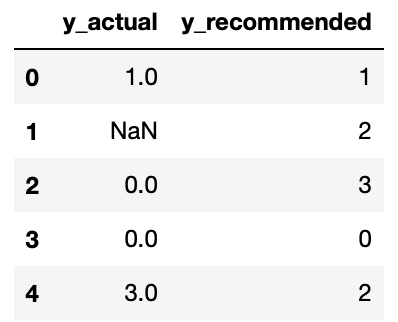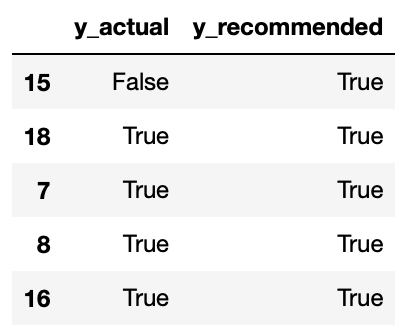For those who prefer a video presentation, you can see me work through the material in this post here:

## What are Precision@k and Recall@K ?

Precision@k and Recall@k are metrics used to evaluate a recommender model. These quantities attempt to measure how effective a recommender is at providing relevant suggestions to users.

The typical workflow of a recommender involves a series of suggestions that will be offered to the end user by the model. Examples of these suggestions could consist of movies to watch, search engine results, potential cases of fraud, etc. These suggestions could number in the 100’s or 1000’s; far beyond the abilities of a typical user to cover in their entirety. The details for how recommenders work is beyond the scope of this article.

Instead of looking at the entire set of possible recommendations, with precision@k and recall@k we’ll only consider the top k suggested items. Before defining these metrics, let’s clarify a few terms:

• Recommended items are items that our model suggests to a particular user
• Relevant items are items that were actually selected by a particular user
• @k, literally read “at k“, where k is the integer number of items we are considering

Now let’s define precision@k and recall@k:

\text{precision@k} = \frac{\text{number of recommended items that are relevant @k}}{\text{number of recommended items @k}}    (1)

\text{recall@k} = \frac{\text{number of recommended items that are relevant @k}}{\text{number of all relevant items}}    (2)

It is apparent that precision@k and recall@k are binary metrics, meaning they are defined in terms of relevant and non-relevant items with respect to the user. They can only be computed for items that a user has actually rated. Precision@k measures what percentage of recommendations produced were actually selected by the user. Conversely, recall@k measures the fraction of all relevant items that were suggested by our model.

In a production setting, recommender performance can be measured with precision@k and recall@k once feedback is obtained on at least k items. Prior to deployment, the recommender can be evaluated on a held-out test set.

## Python Example

Let’s work through a simple example in Python, to illustrate the concepts discussed. We can begin by considering a simple example involving movie recommendations. Imagine we have a pandas dataframe containing actual user movie ratings (y_actual), along with predicted ratings from a recommendation model (y_recommended). These ratings can range from 0.0 (very bad) to 4.0 (very good):

# view the data
df.head(5)Because ratings are an ordeal quantity, we will be primarily interested in the most highly recommended items first. As such, the following preprocessing steps will be required:

1. Sort the dataframe by y_recommended in descending order
2. Remove rows with missing values
3. Convert ratings into a binary quantity (not-relevant, relevant)

We can write some code to implement these steps. I will be considering all ratings of 2.0 or greater to be ‘relevant‘. Conversely, ratings below this threshold will be termed ‘non-relevant‘:

# sort the dataframe
df.sort_values(by='y_recommended',ascending=False,inplace=True)
# remove rows with missing values
df.dropna(inplace=True)
# convert ratings to binary labels
threshold = 2
df = df >= threshold
# view results
df.head(5)Great, we can now work through an implementation of precision@k and recall@k:

def check_inputs(func) -> Callable:
"""
Decorator function to validate inputs to precision_at_k & recall_at_k
"""
def checker(df: pd.DataFrame, k: int=3, y_test: str='y_actual', y_pred: str='y_recommended') -> float:
# check we have a valid entry for k
if k <= 0:
raise ValueError(f'Value of k should be greater than 1, read in as: {k}')
# check y_test & y_pred columns are in df
if y_test not in df.columns:
raise ValueError(f'Input dataframe does not have a column named: {y_test}')
if y_pred not in df.columns:
raise ValueError(f'Input dataframe does not have a column named: {y_pred}')
return func(df, k, y_test, y_pred)
return checker

@check_inputs
def precision_at_k(df: pd.DataFrame, k: int, y_test: str, y_pred: str) -> float:
"""
Function to compute precision@k for an input boolean dataframe

Inputs:
df     -> pandas dataframe containing boolean columns y_test & y_pred
k      -> integer number of items to consider
y_test -> string name of column containing actual user input
y-pred -> string name of column containing recommendation output

Output:
Floating-point number of precision value for k items
"""
# extract the k rows
# compute number of recommended items @k
denominator = dfK[y_pred].sum()
# compute number of recommended items that are relevant @k
numerator = dfK[dfK[y_pred] & dfK[y_test]].shape
# return result
if denominator > 0:
return numerator/denominator
else:
return None

@check_inputs
def recall_at_k(df: pd.DataFrame, k: int, y_test: str, y_pred: str) -> float:
"""
Function to compute recall@k for an input boolean dataframe

Inputs:
df     -> pandas dataframe containing boolean columns y_test & y_pred
k      -> integer number of items to consider
y_test -> string name of column containing actual user input
y-pred -> string name of column containing recommendation output

Output:
Floating-point number of recall value for k items
"""
# extract the k rows
# compute number of all relevant items
denominator = df[y_test].sum()
# compute number of recommended items that are relevant @k
numerator = dfK[dfK[y_pred] & dfK[y_test]].shape
# return result
if denominator > 0:
return numerator/denominator
else:
return None

We can try out these implementations for k = 3, k = 10, and k = 15:

k = 3
print(f'Precision@k: {precision_at_k(df,k):.2f}, Recall@k: {recall_at_k(df,k):.2f} for k={k}')
Precision@k: 0.67, Recall@k: 0.18 for k=3
k = 10
print(f'Precision@k: {precision_at_k(df,k):.2f}, Recall@k: {recall_at_k(df,k):.2f} for k={k}')
Precision@k: 0.80, Recall@k: 0.73 for k=10
k = 15
print(f'Precision@k: {precision_at_k(df,k):.2f}, Recall@k: {recall_at_k(df,k):.2f} for k={k}')
Precision@k: 0.83, Recall@k: 0.91 for k=15

We can see the results for k = 3, k = 10, and k = 15 above. For k = 3, it is apparent that 67% of our recommendations are relevant to the user, and captures 18% of the total relevant items present in the dataset. For k = 10, 80% of our recommendations are relevant to the user, and captures 73% of all relevant items in the dataset. These quantities increase yet further for the k = 15 case.

Note that our sorting and conversion preprocessing steps are only required when dealing with a ordeal label, like ratings. For problems like fraud detection or lead scoring, where the labels are already binary, these steps are not needed. In addition, I have implemented the precision and recall functions to return None if the denominator is 0, since a sensible calculation cannot be made in this case.

These metrics become useful in a live production environment, where capacity to measure the performance of a recommendation model is limited. For example, imagine a scenario where a movie recommender is deployed that can provide 100 movie recommendations per user, per week. However, we are only able to obtain 10 movie ratings from the user during the same time period. In this case, we can measure the weekly performance of our model by computing precision@k and recall@k for k = 10.

### Related Posts